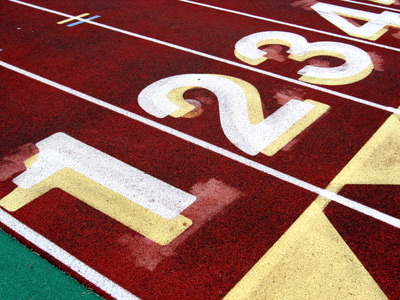1, 2, 3, 4... What number comes next?

# Year 1 Numbers - Number Patterns (Extending)

This quiz addresses the requirements of the National Curriculum KS1 Maths and Numeracy for children aged 5 and 6 in year 1. Specifically this quiz is aimed at the section dealing with recognising and extending number patterns.

Recognising number patterns is taught very early on in KS1. Once children are familiar with the idea that numbers in a sequence do not always increase or decrease by one, they will be asked to extend sequences, or patterns, which may go up or down in twos, fives or tens.

This quiz will help your child to recognise a number pattern and also to be able to extend it.

Question 1
What is the next number in this sequence:
15, 20, 25, 30
53
32
31
35
The numbers are increasing by five each time
Question 2
What is the next number in this sequence?
28, 26, 24, 22
20
21
23
2
The sequence is decreasing by two each time
Question 3
Which number comes next:
35, 40, 45, 50, 55
60 - The numbers are going up in fives
50 - The numbers are going down in fives
57 - The numbers are all odd
56 - The numbers are all even
Each number is five more than the previous one
Question 4
What is the next number in this sequence:
1, 3, 5, 7
8
9
6
10
The numbers are going up in twos, and are all odd
Question 5
Which number comes next in this sequence:
90, 80, 70, 60, 50, 40
35 - The numbers are going down in fives
45 - The numbers are going up in fives
39 - The numbers are going down in ones
30 - The numbers are going down in tens
Each time, the numbers decrease by ten
Question 6
What is the next number in this sequence:
22, 24, 26, 28
32
27
29
30
The numbers are going up in twos each time
Question 7
What is the next number in this sequence:
40, 50, 60, 70
71
69
80
100
The numbers are increasing by ten each time
Question 8
Which number does not belong in the pattern?
23, 25, 26, 27, 29, 31
23
27
26
29
This is the only even number. The others are odd and increasing by two each time
Question 9
Starting at 2, I colour in all the even numbers in order on my hundred square. When I get to 16, which number will I colour in next?
15
17
18
19
18 is the next even number after 16
Question 10
In my sequence, I start at 0, and count up in fives. Which number would be in my sequence?
15
12
13
14
Starting at '0', the sequence would be 0, 5, 10, 15, 20, 25...
Author:  Angela Smith